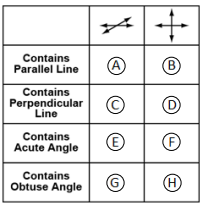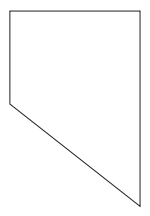# MAFS.4.G.1.1Archived StandardExport Print
Draw points, lines, line segments, rays, angles (right, acute, obtuse), and perpendicular and parallel lines. Identify these in two-dimensional figures.
General Information
Subject Area: Mathematics
Domain-Subdomain: Geometry
Cluster: Level 1: Recall
Cluster: Draw and identify lines and angles, and classify shapes by properties of their lines and angles. (Additional Cluster) -

Clusters should not be sorted from Major to Supporting and then taught in that order. To do so would strip the coherence of the mathematical ideas and miss the opportunity to enhance the major work of the grade with the supporting clusters.

Date of Last Rating: 02/14
Status: State Board Approved - Archived
Assessed: Yes
Test Item Specifications

• Assessment Limits :
s Items may not require students to name a given figure. Items may not require knowledge or use of ordered pairs or a defined coordinate grid system. Items may require students to draw a figure based on multiple attributes (e.g., an acute triangle), with the exception of right triangles. Items that include trapezoids must consider both the inclusive and exclusive definitions. Items may not use the term "kite" but may include the figure.
• Calculator :

No

• Context :

Allowable

Sample Test Items (3)

• Test Item #: Sample Item 2
• Question: Fill in the circles to select all the attributes that apply to each set of lines.• Difficulty: N/A
• Type: MI: Matching Item

• Test Item #: Sample Item 3
• Question: In social studies class, Armando learned about the state of Nevada. He drew the picture shown to represent the shape of Nevada.Which list below correctly describes the kinds of angles that appear to be inside the shape above?

• Difficulty: N/A
• Type: MC: Multiple Choice

## Related Courses

This benchmark is part of these courses.
5012060: Mathematics - Grade Four (Specifically in versions: 2014 - 2015, 2015 - 2022, 2022 and beyond (current))
7712050: Access Mathematics Grade 4 (Specifically in versions: 2014 - 2015, 2015 - 2018, 2018 - 2022, 2022 and beyond (current))
5020110: STEM Lab Grade 4 (Specifically in versions: 2016 - 2022, 2022 and beyond (current))
5012055: Grade 3 Accelerated Mathematics (Specifically in versions: 2019 - 2022, 2022 and beyond (current))
5012015: Foundational Skills in Mathematics 3-5 (Specifically in versions: 2019 - 2022, 2022 and beyond (current))

## Related Access Points

Alternate version of this benchmark for students with significant cognitive disabilities.

## Related Resources

Vetted resources educators can use to teach the concepts and skills in this benchmark.

## Formative Assessments

Students are asked to identify right, acute, and obtuse angles in a two-dimensional figure, and explain the differences among these types of angles.

Type: Formative Assessment

Parallel and Perpendicular Sides:

Students are asked to identify parallel and perpendicular sides and explain how they know.

Type: Formative Assessment

Locating Points, Lines, and Rays:

Students locate points, lines, line segments, and rays in a given diagram.

Type: Formative Assessment

Lines, Rays, and Line Segments:

Students are asked to draw parallel lines, perpendicular lines, a point, and a line segment. Students also explain how a line segment is different from a ray or line.

Type: Formative Assessment

## Lesson Plans

Coding Geometry Challenges #1-7, 14 & 15:

This set of geometry challenges focuses on creating a variety of polygons as students problem solve and think as they learn to code using block coding software.  Student will need to use their knowledge of the attributes of polygons and mathematical principals of geometry to accomplish the given challenges. The challenges start out fairly simple and move to more complex situations in which students can explore at their own pace or work as a team. Computer Science standards are seamlessly intertwined with the math standards while providing “Step it up!” and “Jump it up!” opportunities to increase rigor.

Type: Lesson Plan

Lesson #2 - Moon Phase Unit:

This is lesson 2 of 3 in the Moon Phase unit. This lesson will help students design a flowchart model to find the phase of the Moon by making decisions based on certain conditions. This lesson also gives students insight into working with the design model made earlier and an opportunity to upload/draw costumes of different lunar phases in Scratch.

Type: Lesson Plan

Lesson #1 - Moon Phase Unit :

This is lesson 1 of 3 in the Moon Phase Unit. This lesson introduces students to the eight Moon phases and their names in a counter-clockwise sequential order starting with the New Moon as phase 1. Students will also be introduced to how a flowchart can help make decisions, in this case whether or not the Moon is full.

Type: Lesson Plan

Angles All Around Us:

This lesson will provide students with the opportunity to see angles all around them. Students will be able to see how and where geometry exist in the real world.  Please note that this lesson focuses on identifying acute, obtuse, right, straight and reflex angles.

Type: Lesson Plan

Geometry at the County Fair:

In this lesson which focuses primarily on angles, students work in collaborative groups to construct a two-dimensional model of a county fair.

Type: Lesson Plan

Snowflake Geometry: No Two Alike!:

In this lesson students will make snowflakes, promoting creativity and self-expression, and use them to identify geometric terms. It also gives them an opportunity to follow a sequenced set of instructions for a given outcome.

Type: Lesson Plan

Angles All Around Us:

This is a lesson that introduces right, acute, obtuse, reflex, straight angles in a fun and challenging way.

Type: Lesson Plan

Which Angle is Which?:

This is a fun, hands-on activity designed to help students identify and measure obtuse, acute, right, straight and reflex angles. Students create a manipulative tool in their math journals to help them gain understanding of this concept.

Type: Lesson Plan

Points, Lines, and Angles, Oh My!:

In this lesson, students work to identify points, lines, line segments, rays, angles and perpendicular and parallel lines. Students create webs of yarn and analyze the web for geometric properties listed above. A teacher can select which vocabulary terms are appropriate for the class.

Type: Lesson Plan

A Closer Look at Quadrilaterals - The Parallelogram Connection:

Students work together as a class and in small groups to classify quadrilaterals.

Type: Lesson Plan

Geometry, USA:

Students will draw a town based on a set of given directions using the geometry terms (parallel, perpendicular, and intersecting lines). This activity is designed to be taught after the students having learned the meanings of the geometry terms and the ability to identify examples of each.

Type: Lesson Plan

Runway Rotations:

Students will use small paper airplanes to model rotations required to turn onto a runway. Students will rotate planes 45, 90, 180, 270, and 360 degrees. Students will identify and describe the results of rotations using benchmark angles.

Type: Lesson Plan

## Original Student Tutorials

Shape Surfing: Finding Parallel and Perpendicular Lines:

Help a surfing crab learn how to find parallel and perpendicular sides in a variety of polygons as you complete this interactive tutorial!

Type: Original Student Tutorial

ROC Star: Angle Classification:

Classify and name angles in two-dimensional shapes to help a robot create a path using angles in this interactive tutorial.

Type: Original Student Tutorial

The Geometry Super Heroes Save Mathopolis:

Identify parallel lines and line segments, as well as perpendicular lines and line segments in two-dimensional figures by joining Parallel Man and Perpendicular Man as they help Mayor Mathematics save Mathopolis in this interactive tutorial.

Type: Original Student Tutorial

## Teaching Ideas

Angle Hunting:

In this activity, learners use a hand-made protractor to measure angles they find in playground equipment. Learners will observe that angle measurements do not change with distance, because they are distance invariant, or constant. Note: The "Pocket Protractor" activity should be done ahead as a separate activity (see related resource), but a standard protractor can be used as a substitute.

Type: Teaching Idea

This lesson is designed to introduce students to quadrilaterals and the terms and properties associated with quadrilaterals. This lesson provides links to discussions and activities related to quadrilaterals as well as suggested ways to integrate them into the lesson. Finally, the lesson provides links to follow-up lessons designed for use in succession with the current one.

Type: Teaching Idea

## Tutorials

Parallel and Perpendicular Lines Introduced:

This Khan Academy tutorial video defines and illustrates parallel and perpendicular lines.

Type: Tutorial

Recognizing Acute, Right, and Obtuse Angles:

This Khan Academy tutorial video identifies acute, right, and obtuse angles and justifies each identification.

Type: Tutorial

Lines, Line segments and Rays:

This video discusses the differences between lines, line segments and rays.

Type: Tutorial

## MFAS Formative Assessments

Students are asked to identify right, acute, and obtuse angles in a two-dimensional figure, and explain the differences among these types of angles.

Lines, Rays, and Line Segments:

Students are asked to draw parallel lines, perpendicular lines, a point, and a line segment. Students also explain how a line segment is different from a ray or line.

Locating Points, Lines, and Rays:

Students locate points, lines, line segments, and rays in a given diagram.

Parallel and Perpendicular Sides:

Students are asked to identify parallel and perpendicular sides and explain how they know.

## Original Student Tutorials Mathematics - Grades K-5

ROC Star: Angle Classification:

Classify and name angles in two-dimensional shapes to help a robot create a path using angles in this interactive tutorial.

Shape Surfing: Finding Parallel and Perpendicular Lines:

Help a surfing crab learn how to find parallel and perpendicular sides in a variety of polygons as you complete this interactive tutorial!

The Geometry Super Heroes Save Mathopolis:

Identify parallel lines and line segments, as well as perpendicular lines and line segments in two-dimensional figures by joining Parallel Man and Perpendicular Man as they help Mayor Mathematics save Mathopolis in this interactive tutorial.

## Student Resources

Vetted resources students can use to learn the concepts and skills in this benchmark.

## Original Student Tutorials

Shape Surfing: Finding Parallel and Perpendicular Lines:

Help a surfing crab learn how to find parallel and perpendicular sides in a variety of polygons as you complete this interactive tutorial!

Type: Original Student Tutorial

ROC Star: Angle Classification:

Classify and name angles in two-dimensional shapes to help a robot create a path using angles in this interactive tutorial.

Type: Original Student Tutorial

The Geometry Super Heroes Save Mathopolis:

Identify parallel lines and line segments, as well as perpendicular lines and line segments in two-dimensional figures by joining Parallel Man and Perpendicular Man as they help Mayor Mathematics save Mathopolis in this interactive tutorial.

Type: Original Student Tutorial

## Tutorials

Parallel and Perpendicular Lines Introduced:

This Khan Academy tutorial video defines and illustrates parallel and perpendicular lines.

Type: Tutorial

Recognizing Acute, Right, and Obtuse Angles:

This Khan Academy tutorial video identifies acute, right, and obtuse angles and justifies each identification.

Type: Tutorial

Lines, Line segments and Rays:

This video discusses the differences between lines, line segments and rays.

Type: Tutorial

## Parent Resources

Vetted resources caregivers can use to help students learn the concepts and skills in this benchmark.